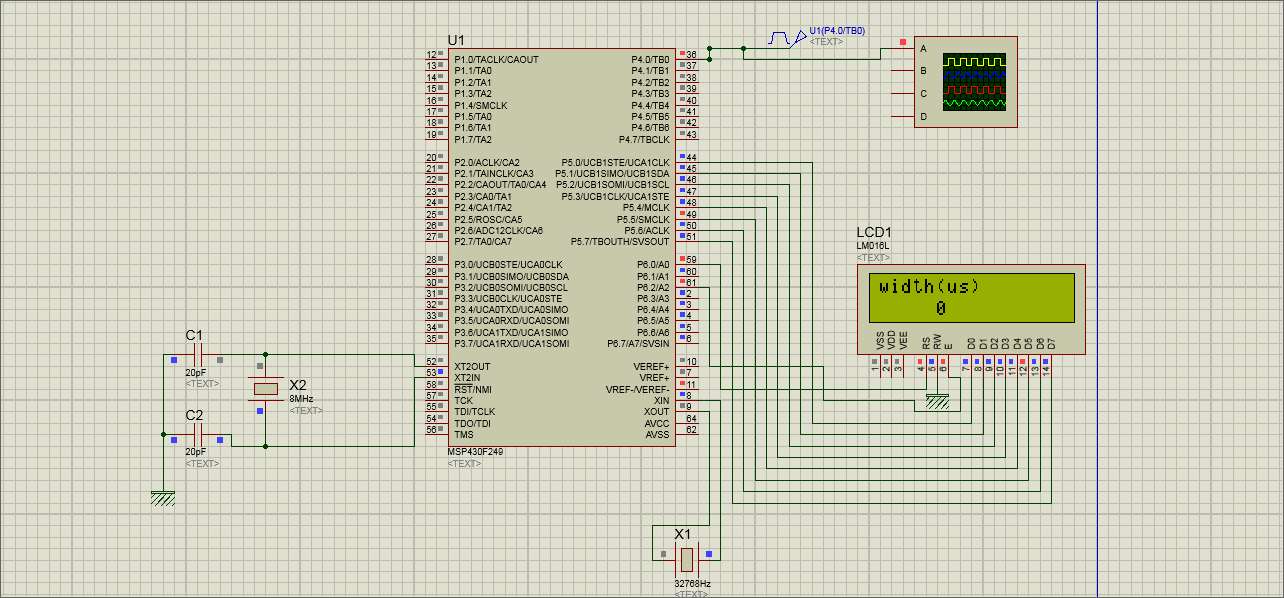main.c文件：
/*定时器B测量某输入信号脉冲宽度*/

#include
#include"lcd.h"
#define M1 10
unsigned int cap1,N1;
long width={0,0,0,0,0,0,0,0,0,0};
char m=0;
char lcd_buf[]={0,0,0,0,0,0};
long data;
const char table[]="0123456789";
const char table1[]="width(us)";
void data_to_buf(unsigned long data2)//值送显示缓冲区函数
{
unsigned char i;
for(i=0;i {
lcd_buf[i]=table[data2%10];//此时低位在前
data2=data2/10;
}
for(i=5;i>0;i--)
{
if(lcd_buf[i]=='0')
lcd_buf[i]=' ';//数字其那面的0不显示
else break;
}
}

void main( void )
{
char num,k1;
// Stop watchdog timer to prevent time out reset
WDTCTL = WDTPW + WDTHOLD;//关闭看门狗

BCSCTL2=SELS+DIVS0+DIVS1;//SMCLK=XT2

P4SEL|=0x01;//P4.0作为捕获模块功能输入端输入方波
P5DIR=0xFF;//设置P5端为输出
P6DIR=0xFF;//设置P6端为输出
lcdinit();
//TBCCTL0=0;//捕获源为P4.0，即CCI0A（也是CCI0B）
TBCCTL0|=CM_1+SCS+CAP+CCIE;//上升沿捕获，同步捕获，工作在捕获模式
//+终端允许
TBCTL|=TBSSEL_2+MC_2+TBIE;//选择SMCLK+连续计数模式
//+终端允许
write_com(0x80);//显示第一行字
for(num=0;num<10;num++)
write_data(table1[num]);
_EINT();//中断允许

while(1)
{
data=0;
for(k1=0;k1<M1;k1++)
data+=width[k1];
data=data/M1;
//data=10000;
if(m==0)
{
data_to_buf(data);//数据送显示缓冲区
write_com(0x80+0x40);//第二行显示频率
for(num=0;num<6;num++)
write_data(lcd_buf[5-num]);
}
}

}

#pragma vector=TIMERB0_VECTOR
__interrupt void TimerB0(void)//定时器TB的CCR0的中断,用于检测脉冲上升与下降
{
if(TBCCTL0&CM1)//捕获到下降沿
{
width[m++]=65536*N1+TBCCR0-cap1;//记录下结束时间
N1=0;
TBCCTL0=CM_1+SCS+CAP+CCIE;//+TBCLR;
//改为上升沿捕获，CM1置0，CM0置1
if(m==M1) m=0;
}
else if(TBCCTL0&CM0)//捕获到上升沿
{
cap1=TBCCR0;
N1=0;
TBCCTL0=CM_2+SCS+CAP+CCIE;//+TBCLR;
//改为下降沿捕获，CM0置0，CM1置1
}
}

//Timer_B7 Interrupt Vector(TBIV) handler
#pragma vector=TIMERB1_VECTOR
__interrupt void Timer_B(void)
{
switch(TBIV)
{
case 14:N1++;break;//溢出
}
}

lcd.c文件：
#include
#define lcdrs_0 P6OUT&=~BIT0;//P6.0=0命令
#define lcdrs_1 P6OUT|=BIT0;//P6.0=1
#define lcden_0 P6OUT&=~BIT2;//P6.2=0
#define lcden_1 P6OUT|=BIT2;//P6.2=1

void delay(unsigned int z)
{
unsigned int i,j;
for(i=z;i>0;i--)
for(j=110;j>0;j--);
}

void write_com(char com) //写入
{
lcdrs_0;//LCD选择输入命令
P5OUT=com;//向P0端口输入命令
delay(5);//延时
lcden_1;//打开LCD使能
delay(5);//一个高脉冲
lcden_0;//关闭LCD使能

}

void write_data(char dataout)
{
lcdrs_1;//设置为输入数据
P5OUT=dataout;//将数据赋给P0端口
delay(5);//延时
lcden_1;//置高
delay(5);//高脉冲
lcden_0;//置低，完成高脉冲
}

void lcdinit()
{
lcden_0;
write_com(0x38);//设置16X2显示5X7点阵，8位数据接口
write_com(0x0c);//设置开始显示，不显示光标
write_com(0x06);//写一个字符后地址指针加1
write_com(0x01);//显示清零，数据指针清零
}

lcd.h文件：
extern void delay(unsigned int z);
extern void write_com(char com);
extern void write_data(char dataout);
extern void lcdinit();1个回答Darlic 回复Iamzhongjiming: 怎么打开晶振？问题解决了吗Iamzhongjiming 这应该是没有打开晶振，我把晶振打开后可以测量占空比了，但测量结果忽大忽小，不知道怎么解决？
5 年多之前 回复